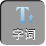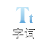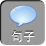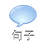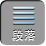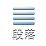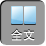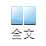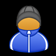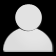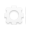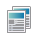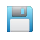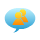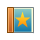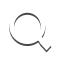-AA+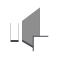1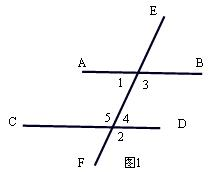∴∠ 5=∠ 2= 110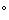（等量代换）

∵∠ 1= 70°（已知）

∴∠ 5+∠ 1= 180AB// CD（同旁内角互补，两直线平行）

∵∠ 1= 70°

∴∠ 3= 110∵∠ 2= 110°

∴∠ 2=∠ 3

AB// CD(同位角相等，两直线平行)

∵∠ 2= 110°

∴∠ 4= 70°

∵∠ 1= 70°

∴∠ 1=∠ 4

AB// CD（内错角相等，两直线平行）

2、如图 2，∠ BAD=∠ BCDCFAE是∠ BCD、∠ BAD的角平分线，∠ 1=∠ 2.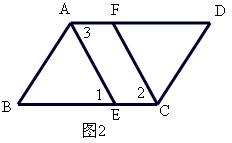∴∠ BCD= 22，∠ BAD= 23（角平分线定义）

∴∠ 2=∠ 3（等量代换）

∴∠ 1=∠ 2（已知）

∴∠ 1=∠ 3（等量代换）

DC// AB(内错角相等，两直线平行)

3、如图 3，已知∠ BED=∠ B+∠ D，试说明 ABCD的位置关系．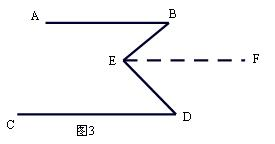EF// AB

∴∠ B=∠ BEF

∵∠ BED=∠ BEF+∠ FED

∴∠ BED=∠ B+∠ FED

∵∠ BED=∠ B+∠ D

∴∠ D=∠ FED

EF// CD

AB// CD(平行于同一直线的两直线平行)

1）如图 4BE平分∠ ABDDE平分∠ BDC，∠ 1+∠ 2= 90，那么直线 ABCD的位置关系如何？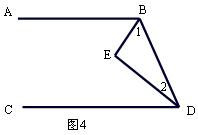2）如图 5，已知∠ B+∠ C+∠ D= 360，则 AB// ED，为什么？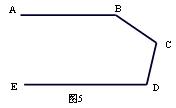1AB// CD

BE平分∠ ABDDE平分∠ BDC

∴∠ ABD= 21，∠ BDC= 22

∴∠ ABD+∠ BDC= 21+ 22

∵∠ 1+∠ 2= 90∴∠ ABD+∠ BDC= 180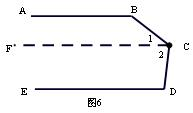AB// CD

2）如图 6，过点 CCF// AB

CF// AB

∴∠ 1+∠ B= 180∵∠ BCD=∠ 1+∠ 2

∵∠ B+∠ BCD+∠ D= 360∴∠ B+∠ 1+∠ 2+∠ D= 360∴∠ 2+∠ D= 180CF// ED

AB// ED